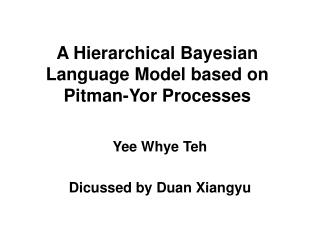# A Hierarchical Bayesian Language Model based on Pitman-Yor Processes - PowerPoint PPT PresentationDownload PresentationA Hierarchical Bayesian Language Model based on Pitman-Yor Processes

A Hierarchical Bayesian Language Model based on Pitman-Yor Processes
Download Presentation## A Hierarchical Bayesian Language Model based on Pitman-Yor Processes

- - - - - - - - - - - - - - - - - - - - - - - - - - - E N D - - - - - - - - - - - - - - - - - - - - - - - - - - -
##### Presentation Transcript

1. A Hierarchical Bayesian Language Model based on Pitman-Yor Processes Yee Whye Teh Dicussed by Duan Xiangyu

2. Introduction • N-gram language model • This paper introduces hierarchical Baysian model for the above, that is, to model • The hierarchical model in this paper is the hierarchical Pitman-Yor processes • Pitman-Yor processes can produce power-law distribution • Hierarchical structure is corresponding to smoothing techniques in language modeling.

3. Introduction of Pitman-Yor Processes • Let W be a vocabulary of V words, G(w) be the probability of a word w, and G=[G(w)]w∈W is the vector of word probabilities. • where base distribution G0=[G0(w)] w∈W, and G0(w)=1/V • d and θ are hyper-parameters.

4. Generative Procedure of PYP • A sequence of words: x1, x2,… drawn i.i.d from G • A sequence of draws y1, y2,… drawn i.i.d from G0 • With probability: , let xc.+1 = yk, that is, next word assigned to previous draw from G0 , letxc.+1 = yt+1, that is, next word assigned to new draw from G0 where t is the current number of draws from G0, ck is the number of words assigned to yk, and . This generative process of PYP exhibits rich get richer phenomenon

5. Metaphor to the Generative Procedure of PYP • Chinese Restaurant Process

6. Hierarchical PYP Language Models • Given context u, let Gu=[Gu(w)]w∈W • π(u) is the suffix of u consisting of all but the earliest word. For example, u is “1 2 3”, then π(u) is “2 3”. • Gπ(u)～PY(d|π(u)|, θ|π(u)|, Gπ(π(u))) • Until Gø～PY(d0, θ0, G0) This is hierarchy

7. Generative Procedure of Hierarchical PYP Language Models • Denotations: • xu1, xu2,… drawn from Gu • yu1, yu2,… drawn from Gπ(u) • We use l to index x, use k to index y. • tuwk=1 if yuk=w • cuwk is the number of words xul=yuk=w • We denote marginal counts by dots • cu.k is the number of words xul=yuk • cuw. is the number of words xul=w • tu.. is the number of draws yuk from Gπ(u)

8. cont.

9. Inference for Hierarchical PYP Language Models • We are interested in predictive probability: • We approximate it with {S(i),θ(i)}i=1I where